# 金拱门戳中笑点？程序员告诉你 起个好名字是成功的一半“麦当劳”、“金拱门”也都呈直线上升的趋势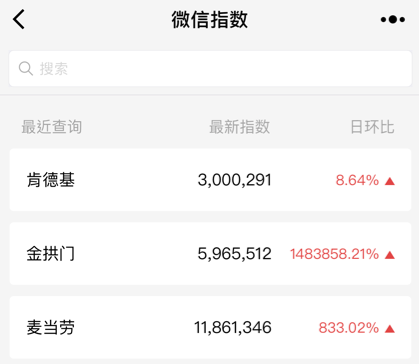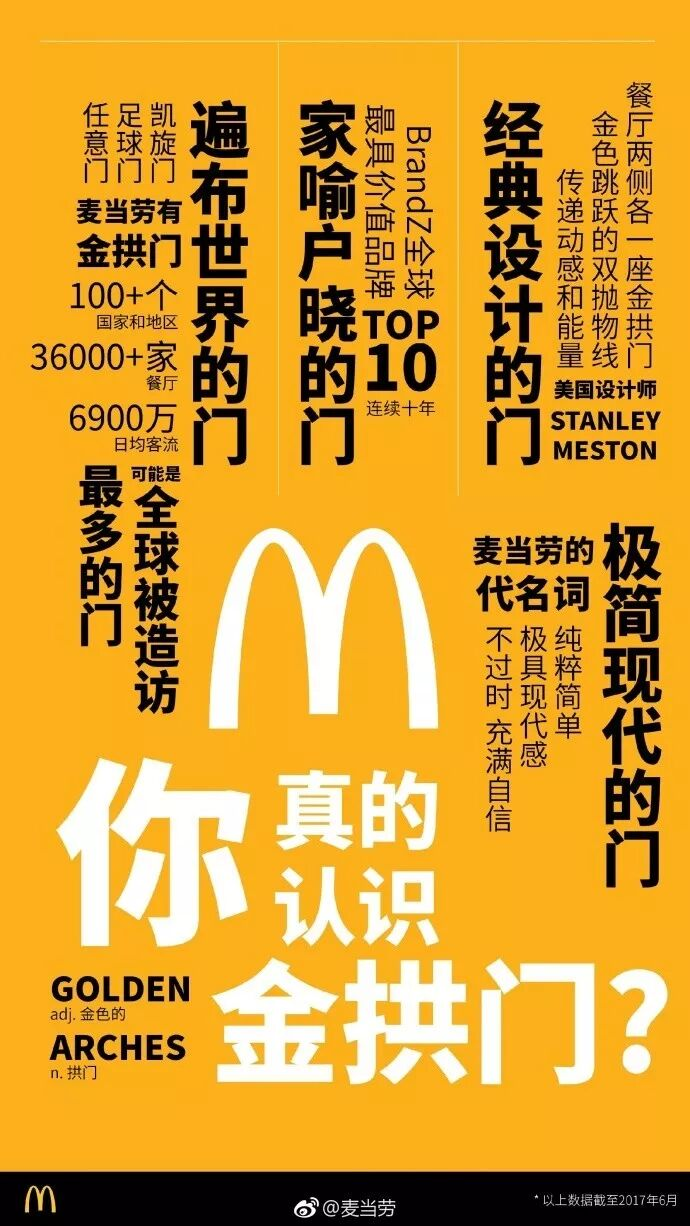BBC曾经就发文表示：“这个世界上最有名的拱门莫过于既没有巧思工匠也没有磅礴历史底蕴的麦当劳。”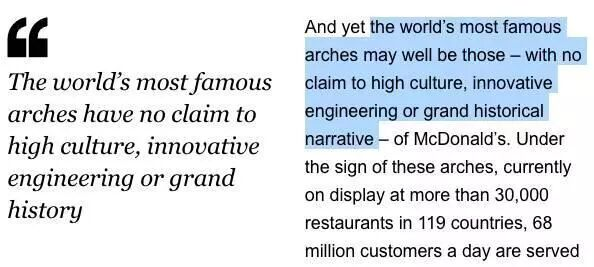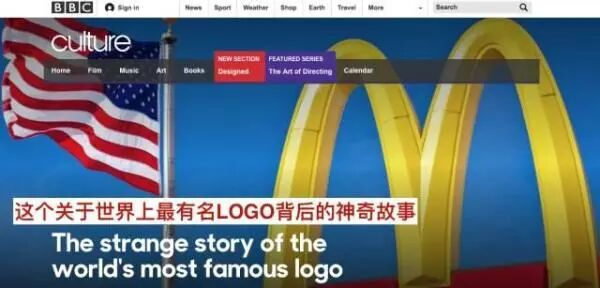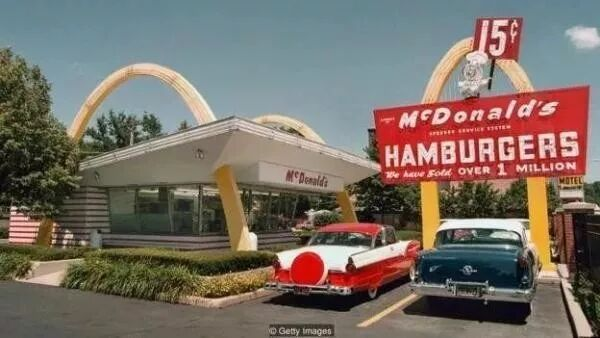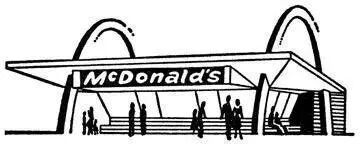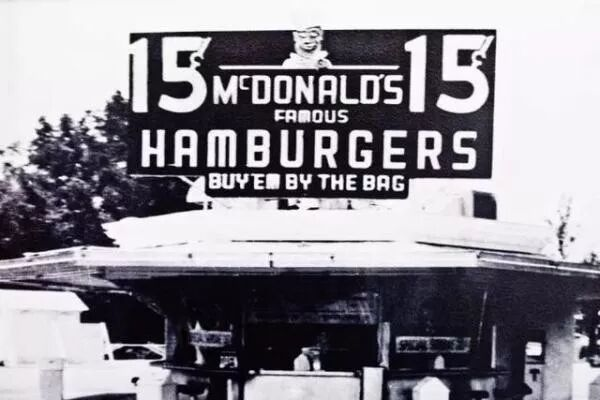1. 没意义的名字

``int a = 1;int b = 2;int c = a + b;System.out.println("c="+c);``

2. 让人误解的名字

``int apple = 1;int banana = 2;int orange = apple  + banana;System.out.println("orange ="+orange);``

3. 数字命名

``int a1 = 1;int a2 = 2;int a3 = 3;int a4 = a1 + a2 ;System.out.println("a4 ="+a4 );``

4. 大家来找茬

``int naturalFirstNum = 1;int naturalSecondNum = 2;int naturalSumNum = naturalFirstNum + naturalSecondNum;System.out.println("naturalSumNum  ="+naturalSumNum );``

5. 不要加和类型相同的名字，这会给你的查找替换工作加大难度

``List<Integer> list = new ArrayList<>();``

``Apple apple = new Apple();``

``Apple mApple = new Apple();``

mApple 比 apple 这个名字好，因为它让 IDE 的查找替换工作更加容易

``int firstNum = 1;int secondNum = 2;int sumNum = firstNum + secondNum;System.out.println("sumNum ="+sumNum );``

``public static void main(String []args){    int firstNum = 1;    int secondNum = 2;    System.out.println("sumNum ="+addTwoNums(firstNum,secondNum ));}public static int addTwoNums(int firstNum,int secondNum){    return firstNum + secondNum;}``

btnCommit，btnModifyPhoneNumber

（总觉得金拱门应该给我们广告费）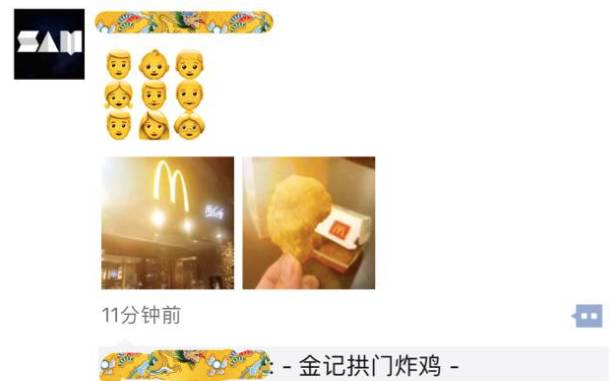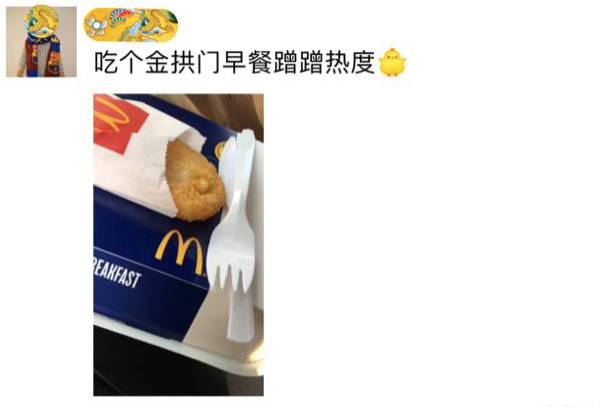2018-09-09

### 谁都有雨天没伞的时候（深度好文）

在晚清的历史上，胡雪岩是家喻户晓的名字。他的成功有很多原因，但他“肯给予别人帮助”的精神也确实令人尊敬。　　一名商人在生意中惨败，需要大笔资金周转。为了救急，他主动[详细]

2017-10-18

2018-08-20

2018-08-01

2018-09-22